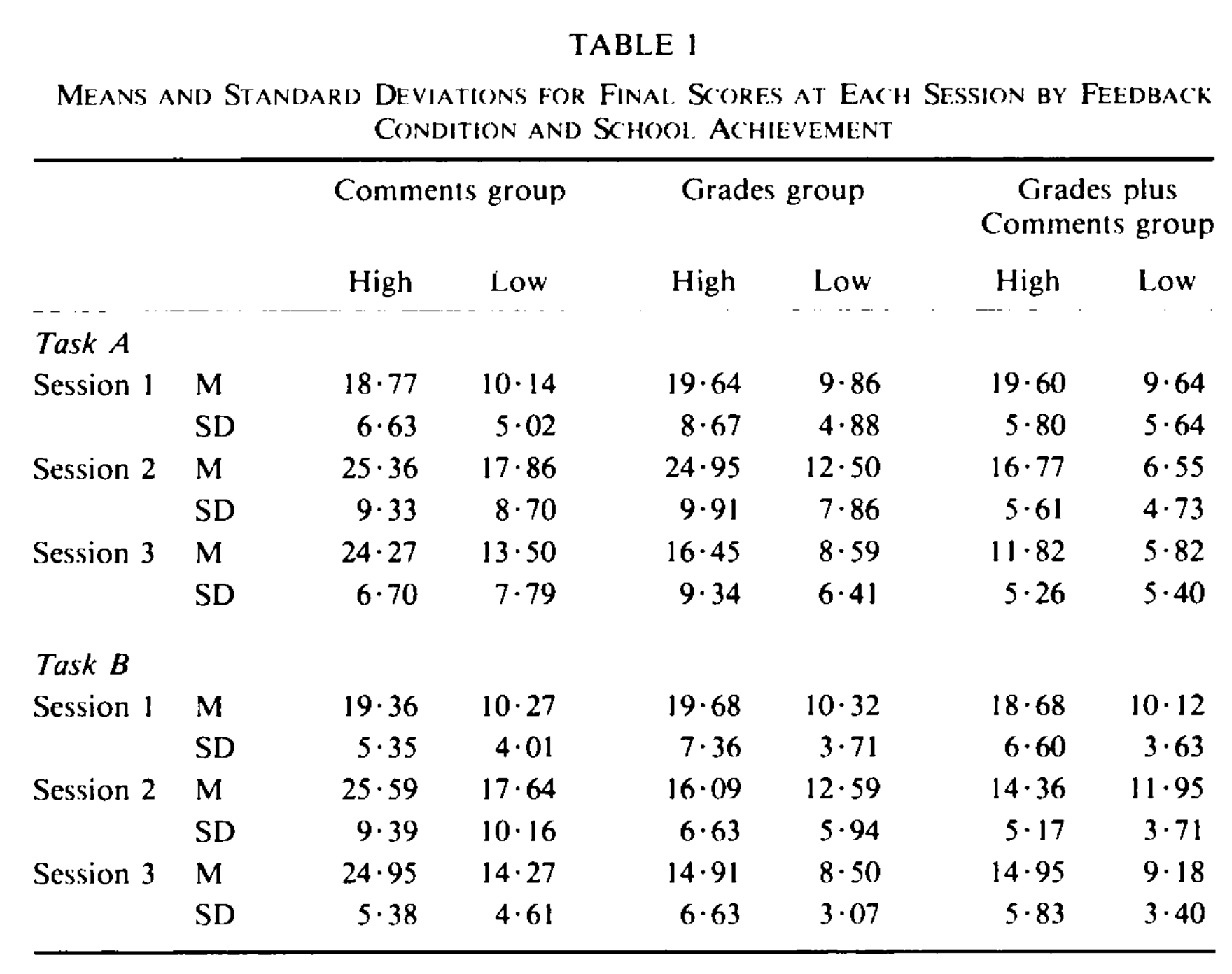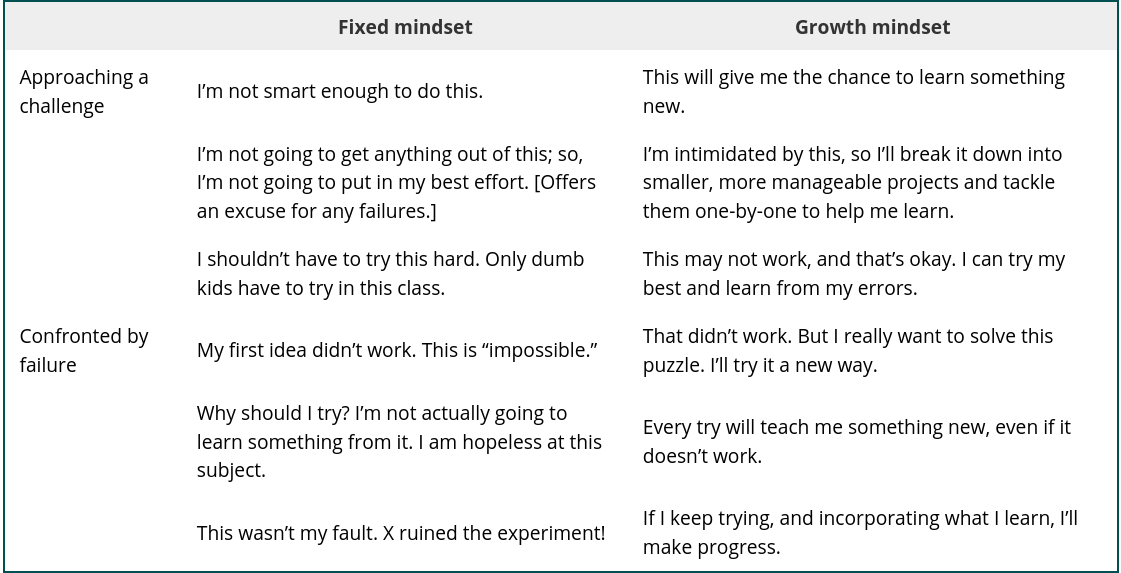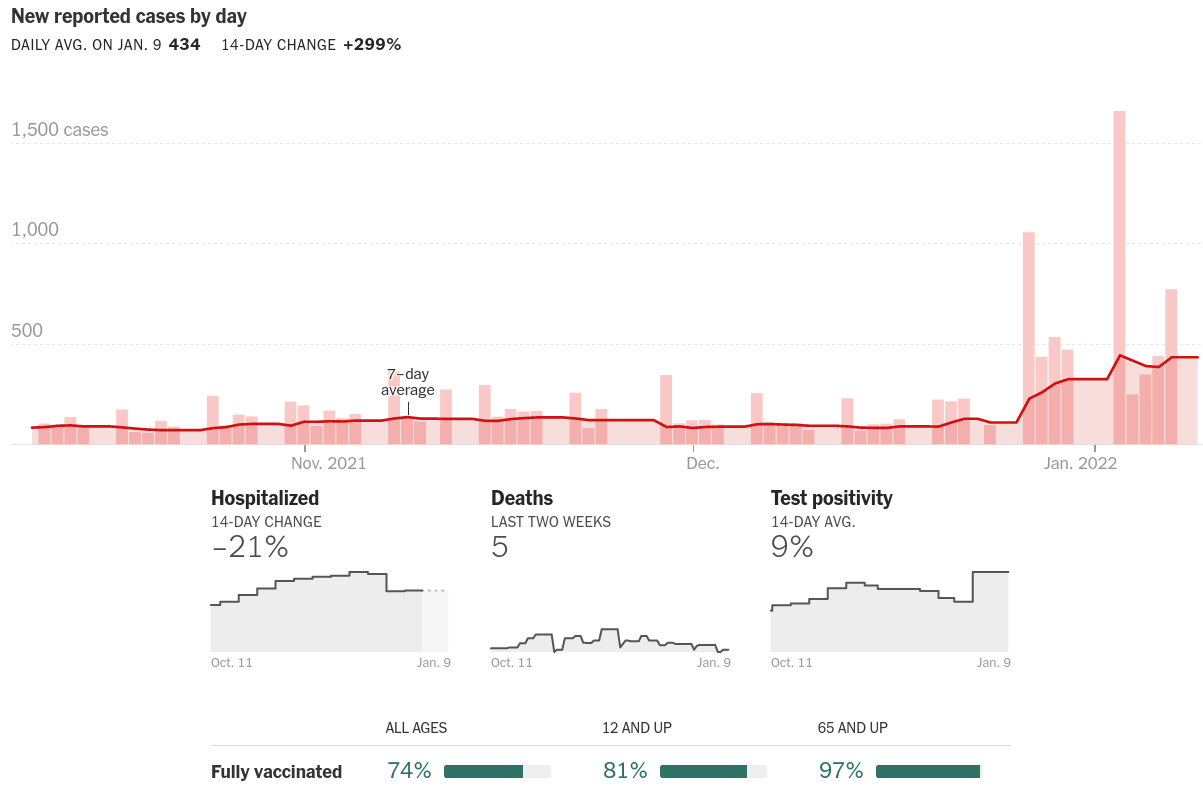# 2022-01-10 First Day¶

## Meeting: Mon, Wed, Fri at 1:25-2:15 in FLMG 157 (and virtually)¶

1. Who am I?

2. What is this course about?

3. How will it work?

4. Discussion

5. (maybe) First activity

# Who am I?¶

• Jed Brown (he/him); you can call me Jed

• Math + Physics undergrad, MS Math, Dr.Sc. Environmental Engineering (Computational Glaciology)

• Postdoc and staff at Argonne National Laboratory (DOE Office of Science)

• CU since 2015

## Physical Prediction, Inference, and Design Group¶

• Develop and maintain open source libraries

• Research in fast/parallel algorithms, extensible software

• Partner with scientists and engineers in many disciplines: geoscience, aerospace, civil engineering, fusion

• Listen, learn about computational bottlenecks, build technology and communities

# Prerequisites¶

## Calculus¶

• Fundamental Theorem of Calculus

• Differentiate and integrate polynomials

• Taylor series

• Gradients (we’ll introduce and discuss)

We won’t use integral tricks (and any time you spent on that was a waste; sorry)

## Linear Algebra¶

• vector spaces, orthogonality

• QR factorization

• projectors, reflectors, and rotations (will teach)

We’ll never compute RREF or eigenvalues by hand (and any time you spent on that was a waste; sorry)

# What is Numerical Computation?¶

From Trefethen (1992)

Here is the wrong answer:

Numerical analysis is the study of rounding errors.

Trefethen goes on to propose

Numerical analysis is the study of algorithms for the problems of continuous mathematics.

## Continuous mathematics¶

• real and complex numbers $$a \in \mathbb R$$

• vectors $$\mathbf x \in \mathbb R^n$$

• matrices $$A \in \mathbb R^{m\times n}$$

• functions $$f: \mathbb R \to \mathbb R$$

• higher order functions: $$g(f)$$

## Finite vs Infinite algorithms¶

• Compute the matrix product $$A B$$

• Solve $$A x = b$$ for $$x$$

• Compute the eigenvalues $$\lambda$$ of $$A \in \mathbb R^{10\times 10}$$

• Compute $$\int_a^b f(x)$$

# Rootfinding¶

Given $$f(x)$$, find $$x$$ such that $$f(x) = 0$$.

• Cost

• Evaluation of $$f(x)$$ and perhaps derivatives $$f'(x)$$.

• Other arithmetic

• Convergence: how many evaluations to reach a given accuracy?

• Robustness: does the algorithm always converge?

using Plots
default(linewidth=3)

f(x) = exp(x) * cos(x) + 1
plot([f, x -> 0], xlims=[-2.5, 5])# Numerical linear algebra¶

## Finite algorithms for factorization¶

• $$QR = A$$, such that $$Q^T Q = I$$ and $$R$$ is right-triangular.

• $$L U = A$$, such that $$L$$ and $$U$$ are lower and upper triangular, respectively.

## Infinite (iterative) algorithms¶

• $$X \Lambda X^{-1} = A$$ where $$\Lambda$$ is diagonal.

• $$U \Sigma V^T = A$$, such that $$U^T U = I$$, $$V^T V = I$$, and $$\Sigma$$ is diagonal with nonnegative entries.

• Iterative solution of $$Ax=b$$ using Krylov basis $$\{b, Ab, A^2b, \dotsc \}$$

## Concepts¶

• Accurate estimates of cost

• Orthogonality, null spaces, projections

• Geometry and modeling

• Stable and backwards-stable algorithms

• Conditioning

• Develop a language in which to frame many concepts in the course

# Approximating functions from data¶

## Interpolation¶

Given samples $$(x_0, f(x_0)), (x_1, f(x_1)), \dotsc$$, determine an easily-computable function $$g(x)$$ that exactly fits these samples and behaves sensibly in between.

## Regression¶

Given noisy data $$(x_0, y_0), (x_1, y_1), \dotsc$$, determine an easily-computable function $$g(x)$$ that explains these data.

We will need to make subjective choices to specify a problem with a unique solution.

# Calculus: functions of functions¶

## Differentiation¶

Given a function $$f(x)$$ (as a computer program or callable function), define a new function

$g(x) \approx f'(x).$

We can do this

• Numerically (black box)

• By-hand code transformation

• Automatic code transformation

## Integration¶

Given a function $$f(x)$$ (as a computer program or callable function), approximate the integral

$\int_a^b f(x) .$

# Numerical optimization¶

Given a scalar function $$f(x)$$ (and perhaps its gradient $$f'(x)$$), determine

$x_* = \operatorname{argmin}_x f(x) .$

# Differential equations¶

Given a dynamical system

$\frac{\partial u(t)}{\partial t} = f(u(t)),$
and initial condition $$u(0) = u_0$$, estimate $$u(t)$$ for arbitrary $$t$$.

# Higher dimensions¶

Many of these algorithms will generalize to functions of multiple variables. Different algorithms may be needed for such “high-dimensional” problems.

# Computer Science = Runnable Abstraction Science¶

## In the “grinder”¶

• Mathematical language and principles for each major class of method

• What is covered by theory

• When you’re venturing off-trail

• Analytic tools to predict and debug

• Realistic cost and exploitable structure

• Performance on modern hardware

• Write/modify stand-alone code

• Select and use (parallel) libraries

## How can you trust the solution?¶

• Verification: solving the problem right

• Validation: solving the right problem

## Abstractions/collaboration¶

• Domain scientists, engineers

• Optimizers, statisticians

• Abstractions that reduce cognitive load

• Metrics/visualization for decisions

# On programming languages¶

## C¶

Reliable and popular for libraries (PETSc, etc.). “Simple”, unsafe, capable of encapsulation.

## C++¶

Popular with applications and some libraries. Powerful, but complicated and unsafe.

## Fortran¶

The OG of numerical computing. Good for “array programming”, but encapsulation is hard. Unsafe depending on dialect.

## Julia¶

For ground-up examples in class and in activities. Capable of high performance, expressive multiple dispatch, works well in a notebook. Error messages are not great.

## Python¶

Good access to JIT, though with sharp edges. Poor native performance, but good libraries to compiled code.

## Rust¶

New compiled language. Good performance, encapsulation, safety, static analysis. Poor libraries (so far).

You don’t need to know any of these well and you can choose the language for your project.# I won’t grade and I won’t waste your time¶

• I will provide feedback

• I will be a mentor and guide navigating this field

• I will help you get what you want out of the class (with a dash of wholesome context)

• My mom said, “basically, your professor is asking you to be an adult”. That was too flexible.

In other classes, I didn’t feel like I could bounce back, but in this one I did.

• Collect a portfolio of the work and insights you’re most proud of

• Individual meetings during the last week of the semester (mid-term preview)

• We’ll have a conversation and you’ll propose a grade based on your portfolio

• I’ll trust you.

I can nudge upward when students are too modest – pretty common. In rare cases, I may adjust down.

# What I need from you: Growth Mindset¶# How will the semester look?¶

## Lecture periods¶

• Refresh, introduce, activity/group discussion, reflect

• Pointers to further resources/activities

## In-class and homework activities¶

• (Short) coding, experiments, presentation of results

• Use the math and programming tools of the field

• Open-ended, go further occasionally

## Learning plans¶

• Write, track, and revise a personal learning plan.

• Meet/chat approx weekly to check in with partner

## Projects (second half of semester)¶

• Dig into community software that implements or relies on numerical computation

• Short presentation on how the community works

• Key stakeholders

• Strengths and weaknesses

• Discuss and critique

## Original study or contribution¶

• Tutorial, documentation, performance study, comparison, new features, new application

• Reflect in a short presentation

# Expectations¶

1. Attend class (in-person or virtual) and participate in discussions

2. Make an honest attempt on activities

3. Interact with the class notebooks and read reference material

4. Set goals and track your progress

• revise these goals as you learn more

1. Meet/chat with your partner weekly for at least a few minutes

• share how lecture and activities are going

• share your goals and how you’re progressing

• identify questions

1. Ask or answer at least one question per week

• ask a question that you don’t know the answer to

• ask a question that you do know the answer to, but you think will be a good exercise/seed for discussion

• ask me during office hours, write up your best understanding

• make a meme relevant to the class

• raise a concern or suggestion that you and/or peers have

• contribute an answer or relevant discussion to any of the above

1. Individual and group projects

# Tools¶

## Git and GitHub¶

• GitHub Classroom to manage repositories

• Write using notebooks and markdown

• Review using GitHub tools and nbgrader

## Zulip¶

• math- and code-aware team chat

• live, informal

• thread managemnet so a question in class can spin off into a deeper asynchronous conversation

## Jupyter¶

• RISE slides available on website

• Activities with scaffolding

• Nbgrader for writing feedback

• Works with many languages; we’ll mostly use Julia

• Collaborative mode (new)

## JupyterHub: coding.csel.io¶

• Nothing to install, persistent storage

• Select CSCI-3656 tile

# A note about COVID¶• Boulder County cases are high and increasing

• Breakthrough cases are very common with Omicron

• I have a 1yo and 4yo in childcare

• Pediatric hospitals are overwhelmed

• They need to stay home and be tested any time they have symptoms• I’d prefer to do virtual office hours until 7-day average new cases drops below 30/day or my child can be vaccinated.

• I’ll likely be home with a kid and need to teach remotely or otherwise make it up to you

# Poll: What is floating point arithmetic?¶

1. fuzzy arithmetic

2. exact arithmetic, correctly rounded

3. the primary focus of numerical analysis

0.1 + 0.2

0.30000000000000004

plot(x -> 1 + x)# Machine epsilon¶

We approximate real numbers with floating point arithmetic, which can only represent discrete values. In particular, there exists a largest number, which we call $$\epsilon_{\text{machine}}$$, such that

$1 \oplus x = 1 \quad \text{for all}\ \lvert x \rvert < \epsilon_{\text{machine}}.$

The notation $$\oplus, \ominus, \odot, \oslash$$ represent the elementary operation carried out in floating point arithmetic.

eps = 1
while 1 + eps != 1
eps = eps / 2
end
eps

1.1102230246251565e-16


# Beating exp¶

$e^x = 1 + x + \frac{x^2}{2!} + \frac{x^3}{3!} + \dotsb$

Suppose we want to compute $$f(x) = e^x - 1$$ for small values of $$x$$.

f1(x) = exp(x) - 1
y1 = f1(1e-8)

9.99999993922529e-9

f2(x) = x + x^2/2 + x^3/6
y2 = f2(1e-8)

1.000000005e-8


Which answer is more accurate?

@show (y1 - y2)        # Absolute difference
@show (y1 - y2) / y2;  # Relative difference

y1 - y2 = -1.1077470910720506e-16
(y1 - y2) / y2 = -1.1077470855333152e-8